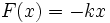# Hooke's law

View other constitutive relations

## Statement

### For springs

Hooke's law for springs states that the magnitude of the restoring force on a spring that is not in its natural length is proportional to the magnitude of the displacement from the natural length, and the direction is opposite the direction of displacement. Thus, if$x$ denotes the displacement from the natural length, the restoring force is given by:$F(x) = -kx$

where$k$ is Hooke's constant (a positive number) and the negative sign is because the direction of the restoring force is opposite to the direction of displacement (if the spring is compressed, it tends to decompress and if the spring is stretched, it tends to shrink back to its natural length).

Hooke's law is valid for displacements of small magnitude for springs.

### More general version

A more general version of Hooke's law states that the magnitude of the restoring force on any body displaced from its natural state is proportional to the magnitude of displacement, and opposite in direction. Hooke's law is not valid in all ranges of displacement but turns out to be a reasonable approximation for small displacements.